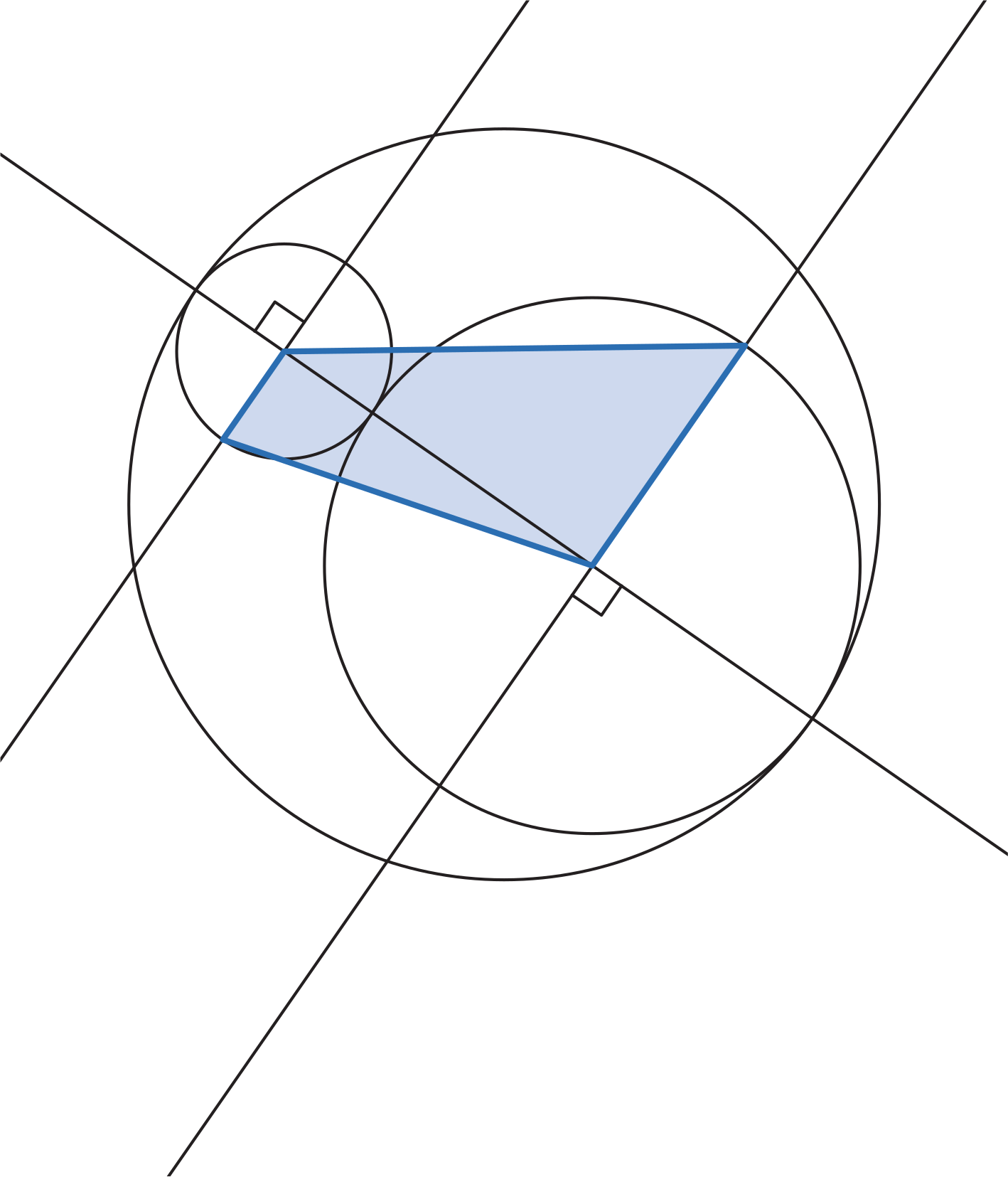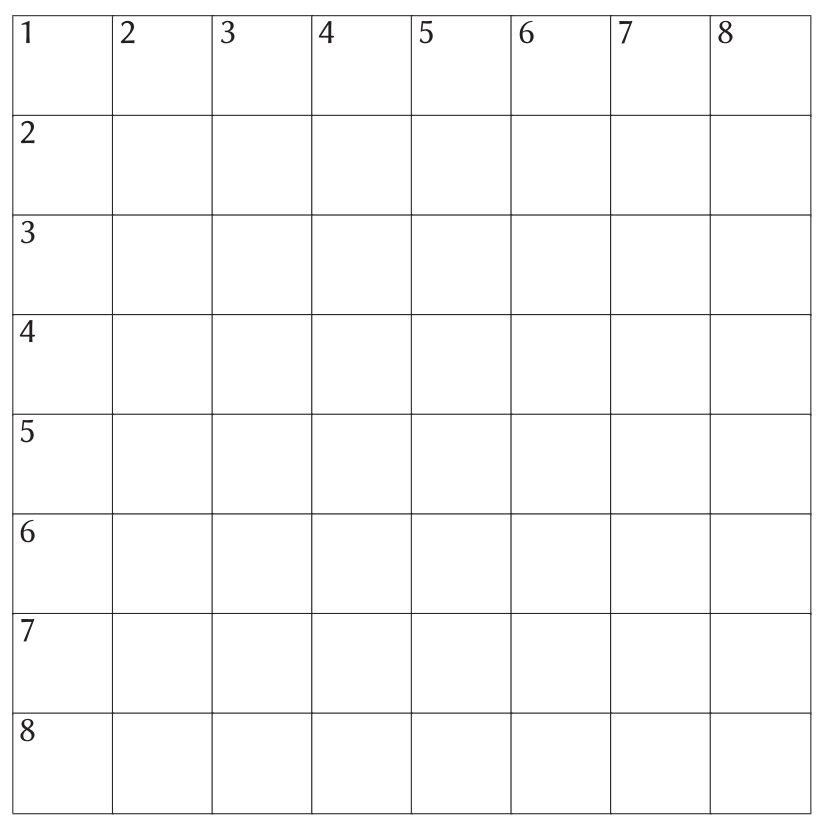# Chalkdust issue 11 puzzle hunt #5

The day is finally here: issue 11 is out now! To help you celebrate launch day in style, we’ve set a puzzle hunt: throughout the day, we are posting a series of puzzles. The answers to these puzzles form clues to the four-digit code for the door to let you into the Chalkdust issue 11 secret backstage lounge.

The fifth and final puzzle is set by Adam Townsend. You can see the puzzle below, or download it as a pdf.

Once you’ve solved this puzzle, head over to the Chalkdust issue 11 secret backstage lounge and unlock the door. See you inside!

Adam has been playing a game: he thinks of a secret word, tells you how many letters long it is, and lets you guess it. For each guess, he tells you how many letters in your guess are the correct letter in the correct position, and how many letters are the correct letter but in the wrong position.

For example, if Adam’s secret word is ‘GAME’, and you guess ‘ELMO’, he will tell you:

 Correct letter,correct position Correct letter,wrong position E L M O 1 1 ? ? ? ?

as the ‘M’ is the correct letter in the correct position, and the ‘E’ is the correct letter in the wrong position. If Adam’s secret word is ‘AABB’ and you guess ‘AAAB’, he will tell you:

 Correct letter,correct position Correct letter,wrong position A A A B 3 0 ? ? ? ?

as three letters are the correct letters in the correct position (AAAB), and there are no letters in the wrong positions as there is no third ‘A’ in Adam’s word. Adam doesn’t double count.

The secret words will spell out a clue to the code.

 Correct letter,correct position Correct letter,wrong position H A T 0 2 E T A 0 2 A R C 0 0 ? ? ?
 Correct letter,correct position Correct letter,wrong position M O D E 3 0 C U B E 2 0 ? ? ? ?
 Correct letter,correct position Correct letter,wrong position G R A D I E N T 1 3 M A N I F O L D 1 3 C O N V E R G E 3 0 N O T A T I O N 2 4 S E Q U E N C E 0 3 M U R D E R E D 0 0 ? ? ? ? ? ? ? ?
 Correct letter,correct position Correct letter,wrong position T A N 1 0 L A W 0 1 O D E 0 1 R O W 0 2 ? ? ?
 Correct letter,correct position Correct letter,wrong position R A N G E 1 1 S H E A R 0 2 I N N E R 2 2 O U T E R 1 0 ? ? ? ? ?# Chalkdust issue 11 puzzle hunt #4

The day is finally here: issue 11 is out now! To help you celebrate launch day in style, we’ve set a puzzle hunt: throughout the day, we are posting a series of puzzles. The answers to these puzzles form clues to the four-digit code for the door to let you into the Chalkdust issue 11 secret backstage lounge.

The fourth puzzle is set by Humbug. You can see the puzzle below, or download it as a pdf.

Be sure to come back at 5pm when the fifth puzzle will be posted.

## Humbug’s puzzle

Although many of the clues have multiple answers, there is only one solution to the completed crossnumber. As usual, no numbers begin with 0.

The number of copies of the digit 1 in the completed crossnumber is one more than a digit of the code.#### Across

• 1 The sum of this number’s digits is 12. (5)
• 5 The middle digit of this number is equal to the first digit of 4D. (3)
• 6 The product of the digits of 5A. (2)
• 7 The sum of this number’s digits is 7. (2)
• 8 1A reversed. (5)

#### Down

• 1 The sum of this number’s digits is 14. (5)
• 2 This number is equal to 2 multiplied by the sum of its digits. (2)
• 3 This number is equal to 3 multiplied by the sum of its digits. (2)
• 4 1D reversed. (5)
• 7 The product of this number’s digits is 7. (2)# Chalkdust issue 11 puzzle hunt #3

The day is finally here: issue 11 is out now! To help you celebrate launch day in style, we’ve set a puzzle hunt: throughout the day, we are posting a series of puzzles. The answers to these puzzles form clues to the four-digit code for the door to let you into the Chalkdust issue 11 secret backstage lounge.

The third puzzle is set by David Sheard. You can see the puzzle below, or download it as a pdf.

Be sure to come back at 3pm when the fourth puzzle will be posted.

## David’s puzzle

The digits 1 to 9 must be entered into the 9×9 grid below following normal sudoku rules: each digit must appear exactly once in each row, column, and 3×3 block. Additionally, the grid also contains thermometers and inequalities.

In each grey thermometer, the number in the circular bulb is the smallest number, and the numbers increase as you move away from the bulb. The sums of the digits on a diagonal indicated by an arrow must satisfy the inequality shown by the arrow (if the sum of the digits on a diagonal is written at the start of the arrow, then the resulting inequality must be true).

In this smaller example puzzle, the digits 1 to 4 must appear in each row, column, and 2×2 block exactly once. Starting from the grey bulbs, the numbers increase along each thermometer; and the numbers in the cells highlighted in blue in the solution add to more than or equal to 7. The sums of the digits on the other
indicated diagonals satisfy the inequalities written by their arrows.The digit in the cell shaded blue is a factor of the code.# Chalkdust issue 11 puzzle hunt #2

The day is finally here: issue 11 is out now! To help you celebrate launch day in style, we’ve set a puzzle hunt: throughout the day, we are posting a series of puzzles. The answers to these puzzles form clues to the code for the door to let you into the Chalkdust issue 11 secret backstage lounge.

The second puzzle is set by TD Dang. You can see the puzzle below, or download it as a pdf.

Be sure to come back at 1pm when the third puzzle will be posted.

## TD’s puzzle

The three circles below all meet at tangents. The area of the largest circle is $20\pi$.

The sum of the first and last digits of the code is less than the area of the blue quadrilateral.# Chalkdust issue 11 puzzle hunt #1

The day is finally here: issue 11 is out now! To help you celebrate launch day in style, we’ve set a puzzle hunt: throughout the day, we are posting a series of puzzles. The answers to these puzzles form clues to the four-digit code for the door to let you into the Chalkdust issue 11 secret backstage lounge.

The first puzzle is set by Matthew Scroggs. You can see the puzzle below, or download it as a pdf.

Be sure to come back at 11am when the second puzzle will be posted.

## Scroggs’s puzzle

Each row and column of this grid contains the letters W, E, T, C, O, I, N, and S exactly once.

Each column and the first 7 rows contain the words given by the clues below in some position. For example, if a row contains the word `WET’, then the row could be WETCOINS,
NOWETSIC, ONSWETCI, or many other things.

Once the puzzle has been completed, the final row will contain a number. This number is one of the digits of the code.#### Across

• 1 Conic ___. (7)
• 2 A charged atom or molecule. (3)
• 3 0 seconds in the future. (3)
• 4 315°. (2)
• 5 3D shape with volume $\pi r^2h/3$. (4)
• 6 225°. (2)
• 7 $\{2,3\}$ or $\emptyset$. (3)

#### Down

• 1 Can be found on page 65 of issue 11. (3)
• 2 $\in$. (2)
• 3 A baby’s favourite trig function (abbrev). (3)
• 4 adjacent ÷ hypotenuse (not abbreviated). (6)
• 5 135°. (2)
• 6 $\sqrt{100}$. (3)
• 7 ___ primes. (4)
• 8 adjacent ÷ hypotenuse (abbrev). (3)